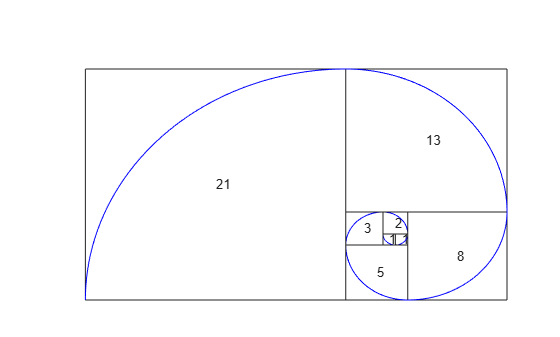# fibonacci

Fibonacci numbers

## Syntax

``fibonacci(n)``

## Description

example

````fibonacci(n)` returns the nth Fibonacci Number.```

## Examples

### Find Fibonacci Numbers

Find the sixth Fibonacci number by using `fibonacci`.

`fibonacci(6)`
```ans = 8```

Find the first 10 Fibonacci numbers.

```n = 1:10; fibonacci(n)```
```ans = 1 1 2 3 5 8 13 21 34 55```

### Fibonacci Sequence Approximates Golden Ratio

The ratio of successive Fibonacci numbers converges to the golden ratio $1.61803...$. Show this convergence by plotting this ratio against the golden ratio for the first 10 Fibonacci numbers.

```n = 2:10; ratio = fibonacci(n)./fibonacci(n-1); plot(n,ratio,'--o') hold on line(xlim,[1.618 1.618]) hold off```### Symbolically Represent Fibonacci Numbers

Use Fibonacci numbers in symbolic calculations by representing them with symbolic input. `fibonacci` returns the input.

Represent the nth Fibonacci number.

```syms n fibonacci(n)```
```ans = fibonacci(n)```

### Find Large Fibonacci Numbers

Find large Fibonacci numbers by specifying the input symbolically using `sym`. Symbolic input returns exact symbolic output instead of double output. Convert symbolic numbers to double by using the `double` function.

Find the 300th Fibonacci number.

```num = sym(300); fib300 = fibonacci(num)```
```fib300 = 222232244629420445529739893461909967206666939096499764990979600```

Convert `fib300` to double. The result is a floating-point approximation.

`double(fib300)`
```ans = 2.2223e+62```

For more information on symbolic and double arithmetic, see Choose Numeric or Symbolic Arithmetic.

### Golden Spiral Using Fibonacci Numbers

The Fibonacci numbers are commonly visualized by plotting the Fibonacci spiral. The Fibonacci spiral approximates the golden spiral.

Approximate the golden spiral for the first 8 Fibonacci numbers. Define the four cases for the right, top, left, and bottom squares in the plot by using a `switch` statement. Form the spiral by defining the equations of arcs through the squares in `eqnArc`. Draw the squares and arcs by using `rectangle` and `fimplicit` respectively.

```x = 0; y = 1; syms v u axis off hold on for n = 1:8 a = fibonacci(n); % Define squares and arcs switch mod(n,4) case 0 y = y - fibonacci(n-2); x = x - a; eqnArc = (u-(x+a))^2 + (v-y)^2 == a^2; case 1 y = y - a; eqnArc = (u-(x+a))^2 + (v-(y+a))^2 == a^2; case 2 x = x + fibonacci(n-1); eqnArc = (u-x)^2 + (v-(y+a))^2 == a^2; case 3 x = x - fibonacci(n-2); y = y + fibonacci(n-1); eqnArc = (u-x)^2 + (v-y)^2 == a^2; end % Draw square pos = [x y a a]; rectangle('Position', pos) % Add Fibonacci number xText = (x+x+a)/2; yText = (y+y+a)/2; text(xText, yText, num2str(a)) % Draw arc interval = [x x+a y y+a]; fimplicit(eqnArc, interval, 'b') end```## Input Arguments

collapse all

Input, specified as a number, vector, matrix or multidimensional array, or a symbolic number, variable, vector, matrix, multidimensional array, function, or expression.

collapse all

### Fibonacci Number

The Fibonacci numbers are the sequence 0, 1, 1, 2, 3, 5, 8, 13, 21….

Given that the first two numbers are 0 and 1, the nth Fibonacci number is

Fn = Fn–1 + Fn–2.

Applying this formula repeatedly generates the Fibonacci numbers.

## Version History

Introduced in R2017a